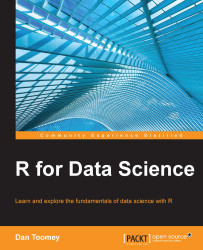•#### R for Data Science#### Overview of this book

R for Data ScienceCreditswww.PacktPub.comPrefaceFree Chapter
Data Mining PatternsData Mining SequencesText MiningData Analysis – Regression AnalysisData Analysis – CorrelationData Analysis – ClusteringData Visualization – R GraphicsData Visualization – PlottingData Visualization – 3DMachine Learning in ActionPredicting Events with Machine LearningSupervised and Unsupervised LearningIndex## Questions

Factual

• What is the best way to handle NA values when performing a regression?

• When will the quantiles graph for a regression model not look like a nice line of fit?

• Can you compare the anova versus manova results? Aside from the multiple sections, is there really a difference in the calculations?

When, how, and why?

• Why does the Residuals vs Leverage graph show such a blob of data?

• Why do we use 4 as a rounding number in the robust regression?

• At what point will you feel comfortable deciding that the dataset you are using for a regression has the right set of predictors in use?

Challenges

• Are there better predictors available for obesity than those used in the chapter?

• How can multilevel regression be used for either the obesity or mpg datasets?

• Can you determine a different set of predictors for mpg that does not reduce it to simple government fiat?